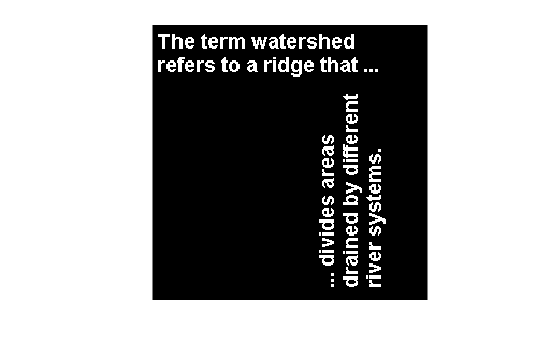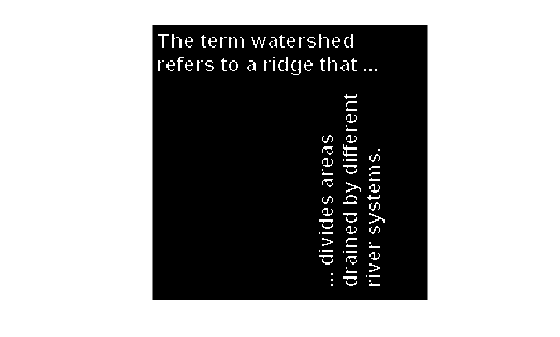# applylut

Neighborhood operations on binary images using lookup tables

`applylut` is not recommended. Use `bwlookup` instead.

## Syntax

``A = applylut(BW,lut)``

## Description

example

````A = applylut(BW,lut)` performs a 2-by-2 or 3-by-3 neighborhood operation on binary image `BW` by using a lookup table, `lut`. The lookup table consists of the output values for all possible 2-by-2 or 3-by-3 neighborhoods.```

## Examples

collapse all

Create the LUT.

``` lutfun = @(x)(sum(x(:))==4); lut = makelut(lutfun,2);```

Read image into the workspace and then apply the LUT to the image. An output pixel is `on` only if all four of the input pixel's neighborhood pixels are `on` .

``` BW1 = imread('text.png'); BW2 = applylut(BW1,lut);```

Show the original image and the eroded image.

` figure, imshow(BW1);`` figure, imshow(BW2);`## Input Arguments

collapse all

Input image, specified as a 2-D binary image. For numeric input, any nonzero pixels are considered to be `1` (`true`).

Data Types: `single` | `double` | `int8` | `int16` | `int32` | `int64` | `uint8` | `uint16` | `uint32` | `uint64` | `logical`

Lookup table of output pixel values, specified as a 16- or 512-element vector as returned by `makelut`.

Data Types: `single` | `double` | `int8` | `int16` | `int32` | `int64` | `uint8` | `uint16` | `uint32` | `uint64` | `logical`

## Output Arguments

collapse all

Output image, returned as a grayscale or binary image whose distribution of pixel values are determined by the content of the lookup table, `lut`. The output image `J` is the same size as the input image `I`.

• If all elements of `lut` are `0` or `1`, then `A` has data type `logical`.

• If all elements of `lut` are integers between 0 and 255, then `A` has data type `uint8`.

• For all other cases, `A` has data type `double`.

Data Types: `double` | `uint8` | `logical`

## Algorithms

collapse all

`applylut` performs a neighborhood operation on a binary image by producing a matrix of indices into `lut`, and then replacing the indices with the actual values in `lut`. The specific algorithm used depends on whether you use 2-by-2 or 3-by-3 neighborhoods.

### 2-by-2 Neighborhoods

For 2-by-2 neighborhoods, `length(lut)` is 16. There are four pixels in each neighborhood, and two possible states for each pixel, so the total number of permutations is 24 = 16.

To produce the matrix of indices, `applylut` convolves the binary image `BW` with this matrix.

```8 2 4 1```

The resulting convolution contains integer values in the range [0, 15]. `applylut` uses the central part of the convolution, of the same size as `BW`, and adds 1 to each value to shift the range to [1, 16]. The function then constructs `A` by replacing the values in the cells of the index matrix with the values in `lut` that the indices point to.

### 3-by-3 Neighborhoods

For 3-by-3 neighborhoods, `length(lut)` is 512. There are nine pixels in each neighborhood, and two possible states for each pixel, so the total number of permutations is 29 = 512.

To produce the matrix of indices, `applylut` convolves the binary image `BW` with this matrix.

```256 32 4 128 16 2 64 8 1```

The resulting convolution contains integer values in the range [0, 511]. `applylut` uses the central part of the convolution, of the same size as `BW`, and adds 1 to each value to shift the range to [1, 512]. It then constructs `A` by replacing the values in the cells of the index matrix with the values in `lut` that the indices point to.

## Compatibility Considerations

expand all

Not recommended starting in R2012b